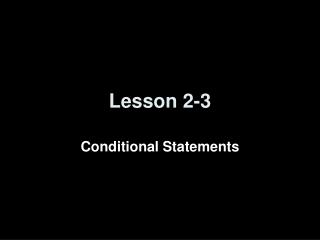Download PresentationLesson 2-3

Loading in 2 Seconds...

# Lesson 2-3 - PowerPoint PPT Presentation

Lesson 2-3. Conditional Statements. Lesson Outline. Five-Minute Check Then & Now and Objectives Vocabulary Key Concept Examples Lesson Checkpoints Summary and Homework. Then and Now.I am the owner, or an agent authorized to act on behalf of the owner, of the copyrighted work described.
Download Presentation## Lesson 2-3

An Image/Link below is provided (as is) to download presentation

Download Policy: Content on the Website is provided to you AS IS for your information and personal use and may not be sold / licensed / shared on other websites without getting consent from its author.While downloading, if for some reason you are not able to download a presentation, the publisher may have deleted the file from their server.

- - - - - - - - - - - - - - - - - - - - - - - - - - E N D - - - - - - - - - - - - - - - - - - - - - - - - - -
Presentation Transcript
1. Lesson 2-3 Conditional Statements

2. Lesson Outline • Five-Minute Check • Then & Now and Objectives • Vocabulary • Key Concept • Examples • Lesson Checkpoints • Summary and Homework

3. Then and Now You used logic and Venn diagrams to determine truth values of negations, conjunctions, and disjunctions. (Lesson 2–2) • Analyze statements in if-then form. • Write the converse, inverse, and contrapositive of if-then statements.

4. Objectives • Analyze statements in if-then form • Write the converse, inverse and contrapositive of if-then statements

5. Vocabulary • Implies symbol(→) • Conditional statement – a statement written in if-then form • Hypothesis – phrase immediately following the word “if” in a conditional statement • Conclusion – phrase immediately following the word “then” in a conditional statement • Converse – exchanges the hypothesis and conclusion of the conditional statement • Inverse – negates both the hypothesis and conclusion of the conditional statement • Contrapositive – negates both the hypothesis and conclusion of the converse statement • Logically equivalent – multiple statements with the same truth values • Biconditional – conjunction of the conditional and its converse

6. Key Concept

7. hypothesis conclusion Example 1A A. Identify the hypothesis and conclusion of the following statement. If a polygon has 6 sides, then it is a hexagon. If a polygon has 6 sides, then it is a hexagon. Answer:Hypothesis: A polygon has 6 sides.Conclusion: It is a hexagon.

8. Example 1B B.Identify the hypothesis and conclusion of the following statement. Tamika will advance to the next level of play if she completes the maze in her computer game. Answer:Hypothesis: Tamika completes the maze in her computer game.Conclusion: She will advance to the next level of play.

9. Example 2A A. Identify the hypothesis and conclusion of the following statement. Then write the statement in the if-then form. Measured distance is positive. Answer:Hypothesis: A distance is measured.Conclusion: It is positive.If a distance is measured, then it is positive.

10. Example 2B B. Identify the hypothesis and conclusion of the following statement. Then write the statement in the if-then form. A five-sided polygon is a pentagon. Answer:Hypothesis: A polygon has five sides.Conclusion: It is a pentagon.If a polygon has five sides, then it is a pentagon.

11. Example 3A A. Determine the truth value of the conditional statement. If true, explain your reasoning. If false, give a counterexample. If you subtract a whole number from another whole number, the result is also a whole number. Counterexample: 2 – 7 = –5 2 and 7 are whole numbers, but –5 is an integer, not a whole number. The conclusion is false. Answer:Since you can find a counterexample, the conditional statement is false.

12. Example 3B B. Determine the truth value of the conditional statement. If true, explain your reasoning. If false, give a counterexample. If last month was February, then this month is March. When the hypothesis is true, the conclusion is also true, since March is the month that follows February. Answer:So, the conditional statement is true.

13. Example 3C • Determine the truth value of the conditional statement. If true, explain your reasoning. If false, give a counterexample. • When a rectangle has an obtuse angle, it is a parallelogram. The hypothesis is false, since a rectangle can never have an obtuse angle. A conditional with a false hypothesis is always true. Answer:So, the conditional statement is true.

14. Key Concept

15. Key Concept

16. Example 4A NATURE Write the converse, inverse, and contrapositive of the following true statement. Determine the truth value of each statement. If a statement is false, give a counterexample. Bats are animals that can fly. Bats are not birds, they are mammals. Bats have modified hands and arms that serve as wings. They are the only mammals that can fly. Conditional:First, rewrite the conditional in if-then form. If an animal is a bat, then it can fly. This statement is true.

17. Example 4 cont Converse:If an animal can fly, then it is a bat. Counterexample: A bird is an animal that can fly, but it is not a bat. The converse is false. Inverse:If an animal is not a bat, then it cannot fly. Counterexample: A bird is not a bat, but it is an animal that can fly. The inverse is false. Contrapositive:If an animal cannot fly, then it is not a bat. The converse is true.

18. Lesson Checkpoints

19. Conditionals in Symbols

20. Summary & Homework • Summary: • A polygon is a closed figure made of line segments • The perimeter of a polygon is the sum of the lengths of its sides • The perimeter of a circle is called the circumference • The area of a two-dimensional figure can be determined by formulas on the formula sheet • Homework: • pg 70-3: 18-23